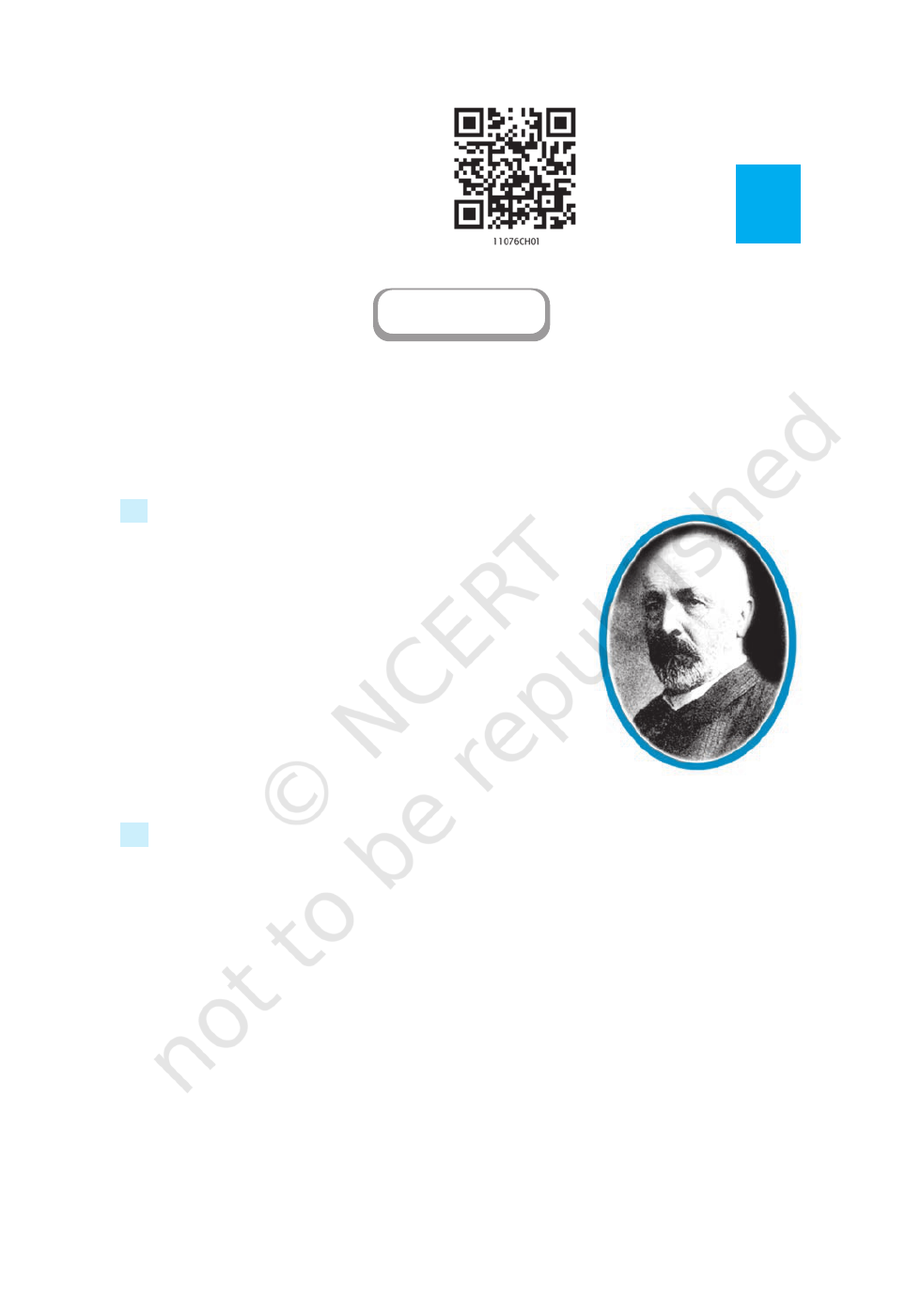Chapter 1
SETS
Georg Cantor
(1845-1918)
vIn these days of conflict between ancient and modern studies; there
must surely be something to be said for a study which did not
begin with Pythagoras and will not end with Einstein; but
is the oldest and the youngest. — G.H. HARDY v
1.1 Introduction
The concept of set serves as a fundamental part of the
present day mathematics. Today this concept is being used
in almost every branch of mathematics. Sets are used to
define the concepts of relations and functions. The study of
geometry, sequences, probability, etc. requires the knowledge
of sets.
The theory of sets was developed by German
mathematician Georg Cantor (1845-1918). He first
encountered sets while working on “problems on trigonometric
series”. In this Chapter, we discuss some basic definitions
and operations involving sets.
1.2 Sets and their Representations
In everyday life, we often speak of collections of objects of a particular kind, such as,
a pack of cards, a crowd of people, a cricket team, etc. In mathematics also, we come
across collections, for example, of natural numbers, points, prime numbers, etc. More
specially, we examine the following collections:
(i) Odd natural numbers less than 10, i.e., 1, 3, 5, 7, 9
(ii) The rivers of India
(iii) The vowels in the English alphabet, namely, a, e, i, o, u
(iv) Various kinds of triangles
(v) Prime factors of 210, namely, 2,3,5 and 7
(vi) The solution of the equation: x
2
– 5x + 6 = 0, viz, 2 and 3.
We note that each of the above example is a well-defined collection of objects in
2020-21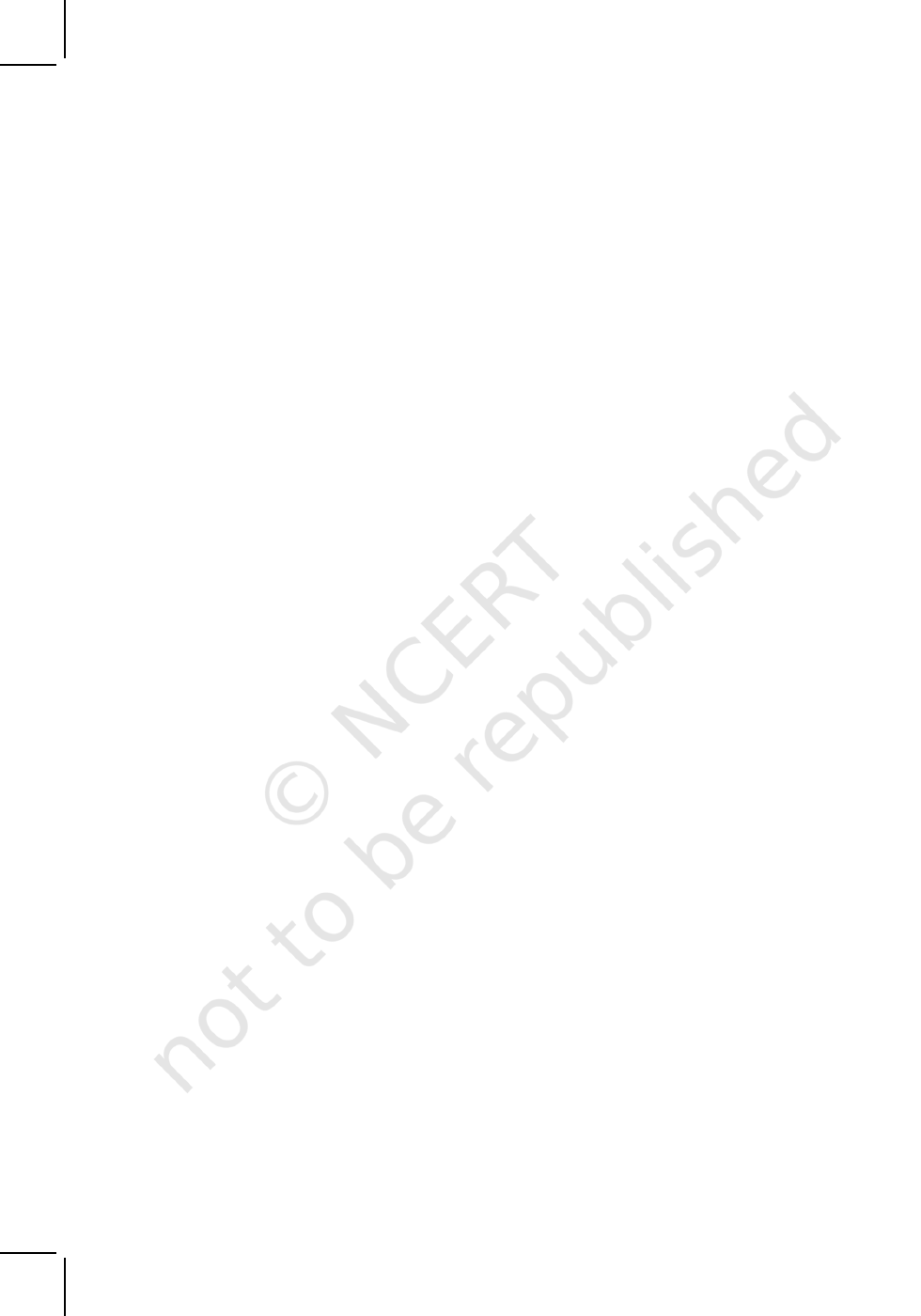2 MATHEMATICS
the sense that we can definitely decide whether a given particular object belongs to a
given collection or not. For example, we can say that the river Nile does not belong to
the collection of rivers of India. On the other hand, the river Ganga does belong to this
colleciton.
We give below a few more examples of sets used particularly in mathematics, viz.
N : the set of all natural numbers
Z : the set of all integers
Q : the set of all rational numbers
R : the set of real numbers
Z
+
: the set of positive integers
Q
+
: the set of positive rational numbers, and
R
+
: the set of positive real numbers.
The symbols for the special sets given above will be referred to throughout
this text.
Again the collection of five most renowned mathematicians of the world is not
well-defined, because the criterion for determining a mathematician as most renowned
may vary from person to person. Thus, it is not a well-defined collection.
We shall say that a set is a well-defined collection of objects.
The following points may be noted :
(i) Objects, elements and members of a set are synonymous terms.
(ii) Sets are usually denoted by capital letters A, B, C, X, Y, Z, etc.
(iii) The elements of a set are represented by small letters a, b, c, x, y, z, etc.
If a is an element of a set A, we say that “ a belongs to A” the Greek symbol
(epsilon) is used to denote the phrase ‘belongs to
’. Thus, we write a A. If ‘b is not
an element of a set A, we write b A and read “b does not belong to A”.
Thus, in the set V of vowels in the English alphabet, a V but b
V. In the set
P of prime factors of 30, 3 P but 15 P.
There are two methods of representing a set :
(i) Roster or tabular form
(ii) Set-builder form.
(i) In roster form, all the elements of a set are listed, the elements are being separated
by commas and are enclosed within braces { }. For example, the set of all even
positive integers less than 7 is described in roster form as {2, 4, 6}. Some more
examples of representing a set in roster form are given below :
(a) The set of all natural numbers which divide 42 is {1, 2, 3, 6, 7, 14, 21, 42}.
2020-21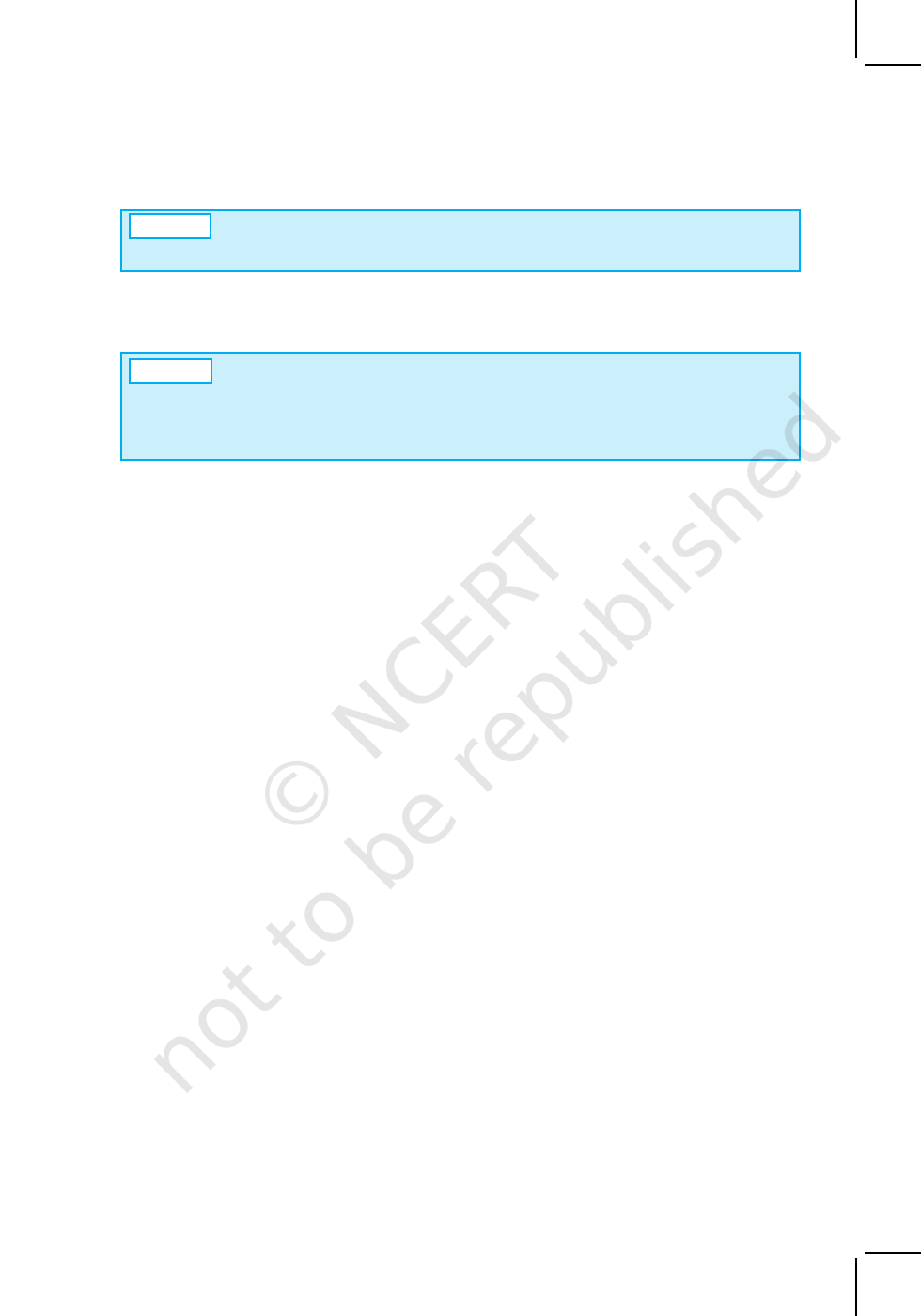SETS 3
A
Note In roster form, the order in which the elements are listed is immaterial.
Thus, the above set can also be represented as {1, 3, 7, 21, 2, 6, 14, 42}.
(b) The set of all vowels in the English alphabet is {a, e, i, o, u}.
(c) The set of odd natural numbers is represented by {1, 3, 5, . . .}. The dots
tell us that the list of odd numbers continue indefinitely.
A
Note It may be noted that while writing the set in roster form an element is not
generally repeated, i.e., all the elements are taken as distinct. For example, the set
of letters forming the word ‘SCHOOL’ is { S, C, H, O, L} or {H, O, L, C, S}. Here,
the order of listing elements has no relevance.
(ii) In set-builder form, all the elements of a set possess a single common property
which is not possessed by any element outside the set. For example, in the set
{
a, e, i, o, u}, all the elements possess a common property, namely, each of them
is a vowel in the English alphabet, and no other letter possess this property. Denoting
this set by V, we write
V = {x : x is a vowel in English alphabet}
It may be observed that we describe the element of the set by using a symbol x
(any other symbol like the letters y, z, etc. could be used) which is followed by a colon
“ : ”. After the sign of colon, we write the characteristic property possessed by the
elements of the set and then enclose the whole description within braces. The above
description of the set V is read as “the set of all x such that x is a vowel of the English
alphabet”. In this description the braces stand for “the set of all”, the colon stands for
“such that”. For example, the set
A = {x : x is a natural number and 3 < x < 10} is read as “the set of all x such that
x is a natural number and x lies between 3 and 10.” Hence, the numbers 4, 5, 6,
7, 8 and 9 are the elements of the set A.
If we denote the sets described in (a), (b) and (c) above in roster form by A, B,
C, respectively, then A, B, C can also be represented in set-builder form as follows:
A= {x : x is a natural number which divides 42}
B= {y : y is a vowel in the English alphabet}
C= {z : z is an odd natural number}
Example 1 Write the solution set of the equation x
2
+ x – 2 = 0 in roster form.
Solution
The given equation can be written as
(x – 1) (x + 2) = 0, i. e., x = 1, – 2
Therefore, the solution set of the given equation can be written in roster form as {1, – 2}.
Example 2
Write the set {
x : x is a positive integer and x
2
< 40} in the roster form.
2020-21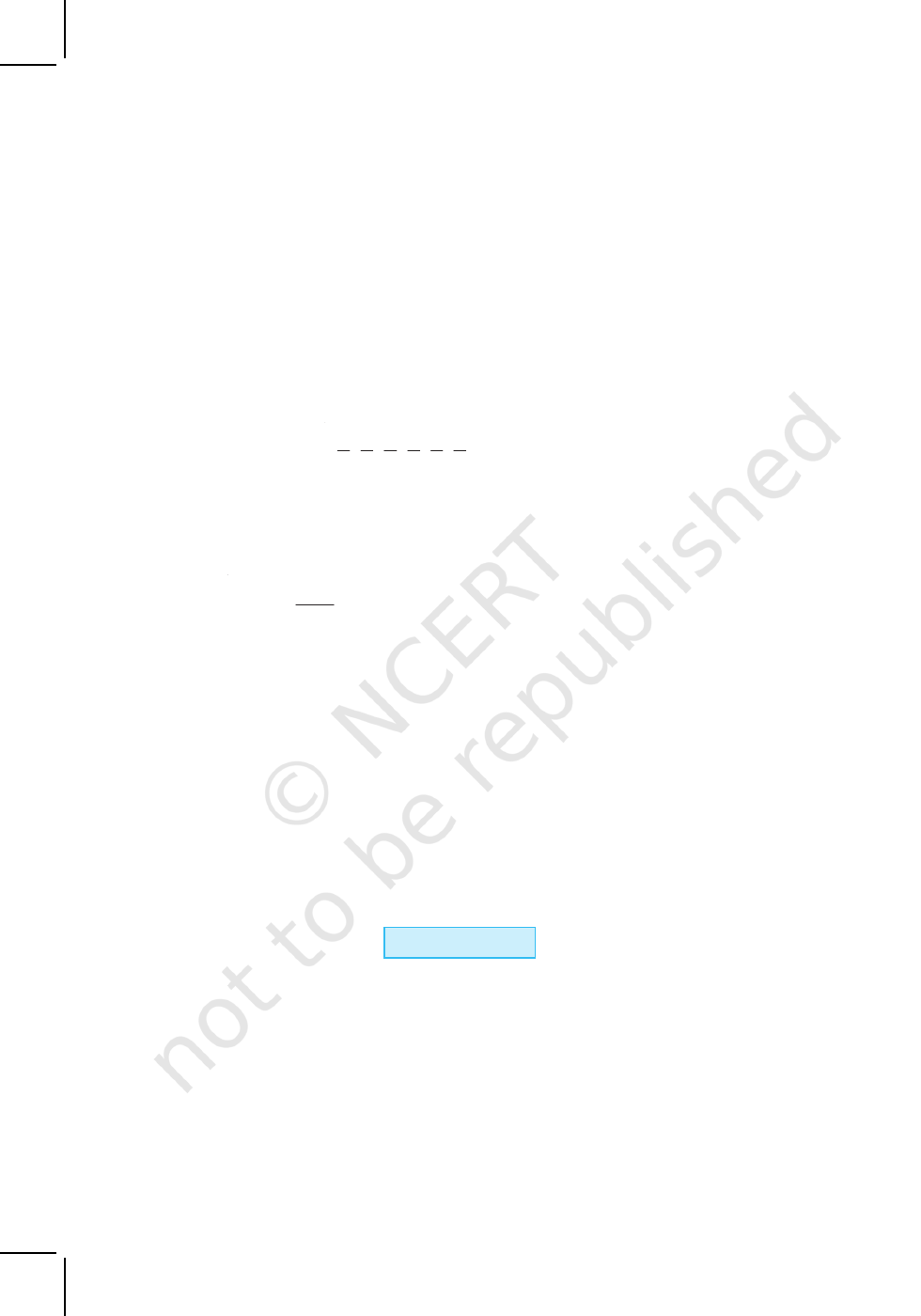4 MATHEMATICS
Solution The required numbers are 1, 2, 3, 4, 5, 6. So, the given set in the roster form
is {1, 2, 3, 4, 5, 6}.
Example 3 Write the set A = {1, 4, 9, 16, 25, . . . }in set-builder form.
Solution
We may write the set A as
A = {x : x is the square of a natural number}
Alternatively, we can write
A = {x : x = n
2
, where n N}
Example 4
Write the set
1 2 3 4 5 6
{ }
2 3 4 5 6 7
, , , , ,
in the set-builder form.
Solution We see that each member in the given set has the numerator one less than
the denominator. Also, the numerator begin from 1 and do not exceed 6. Hence, in the
set-builder form the given set is
where is a natural number and 1 6
1
n
x : x , n n
n
=
+
Example 5 Match each of the set on the left described in the roster form with the
same set on the right described in the set-builder form :
(i) {P, R, I, N, C, A, L} (a) { x : x is a positive integer and is a divisor of 18}
(ii) { 0 } (b) { x : x is an integer and x
2
– 9 = 0}
(iii) {1, 2, 3, 6, 9, 18} (c) {x : x is an integer and x + 1= 1}
(iv) {3, –3} (d) {x : x is a letter of the word PRINCIPAL}
Solution Since in (d), there are 9 letters in the word PRINCIPAL and two letters P and I
are repeated, so (i) matches (d). Similarly, (ii) matches (c) as x + 1 = 1 implies
x = 0. Also, 1, 2 ,3, 6, 9, 18 are all divisors of 18 and so (iii) matches (a). Finally, x
2
– 9 = 0
implies x = 3, –3 and so (iv) matches (b).
EXERCISE 1.1
(i) The collection of all the months of a year beginning with the letter J.
(ii) The collection of ten most talented writers of India.
(iii) A team of eleven best-cricket batsmen of the world.
(iv) The collection of all boys in your class.
(v) The collection of all natural numbers less than 100.
(vi) A collection of novels written by the writer Munshi Prem Chand.
(vii) The collection of all even integers.
2020-21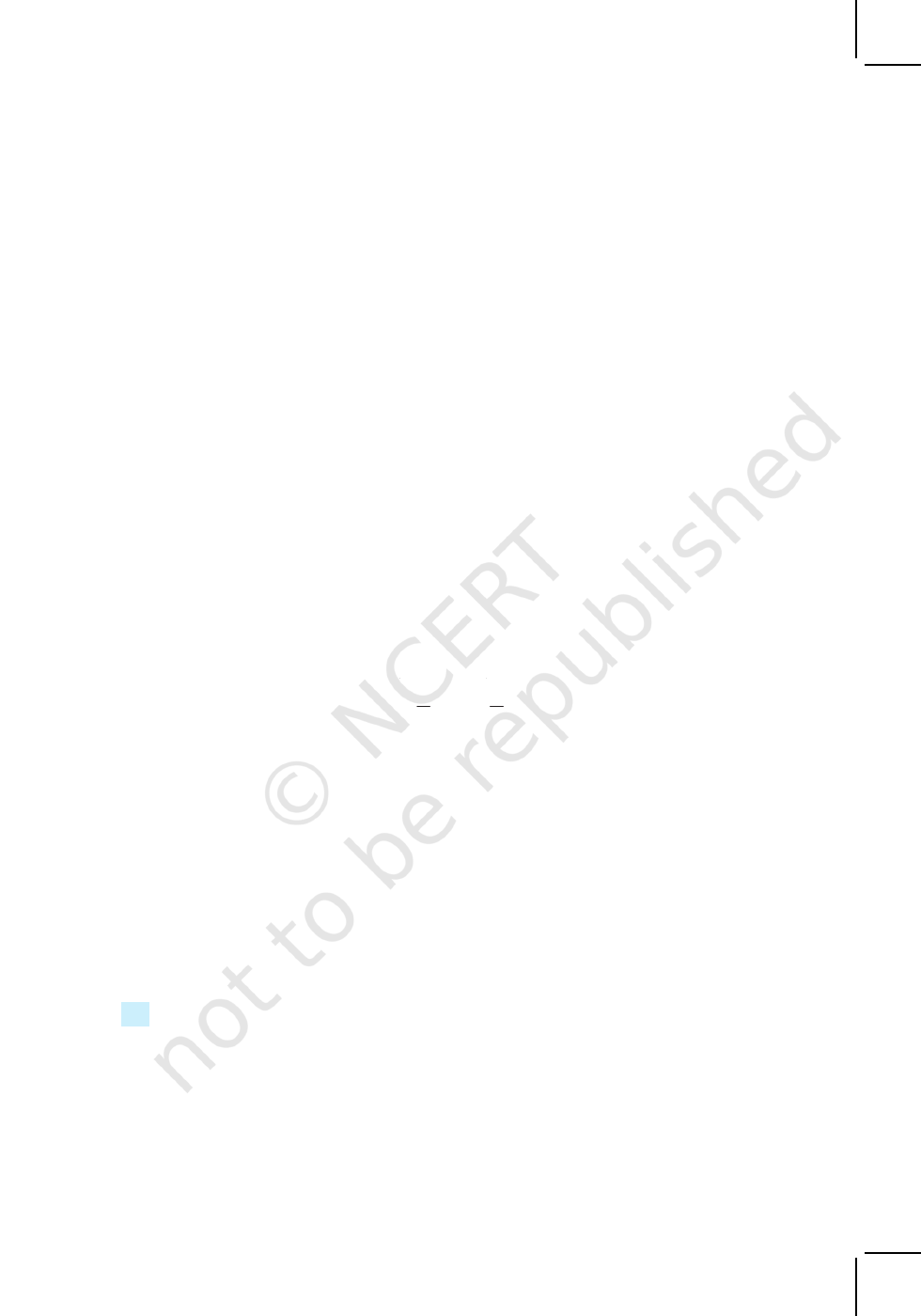SETS 5
(viii) The collection of questions in this Chapter.
(ix) A collection of most dangerous animals of the world.
2. Let A = {1, 2, 3, 4, 5, 6}. Insert the appropriate symbol or in the blank
spaces:
(i) 5. . .A (ii) 8 . . . A (iii) 0. . .A
(iv) 4. . . A (v) 2. . .A (vi) 10. . .A
3. Write the following sets in roster form:
(i) A = {x : x is an integer and –3 x < 7}
(ii) B = {x
: x
is a natural number less than 6}
(iii) C = {x : x is a two-digit natural number such that the sum of its digits is 8}
(iv) D = {x : x is a prime number which is divisor of 60}
(v) E = The set of all letters in the word TRIGONOMETRY
(vi) F = The set of all letters in the word BETTER
4. Write the following sets in the set-builder form :
(i) (3, 6, 9, 12} (ii) {2,4,8,16,32} (iii) {5, 25, 125, 625}
(iv) {2, 4, 6, . . .} (v) {1,4,9, . . .,100}
5. List all the elements of the following sets :
(i) A = {
x : x is an odd natural number}
(ii) B = {x : x is an integer,
2
< x <
9
2
}
(iii) C = {x : x is an integer, x
2
4}
(iv) D = {x : x is a letter in the word “LOYAL”}
(v) E = {x : x is a month of a year not having 31 days}
(vi) F = {x : x is a consonant in the English alphabet which precedes k }.
6. Match each of the set on the left in the roster form with the same set on the right
described in set-builder form:
(i) {1, 2, 3, 6} (a) {
x : x is a prime number and a divisor of 6}
(ii) {2, 3} (b) {x : x is an odd natural number less than 10}
(iii) {M,A,T,H,E,I,C,S} (c) {x : x is natural number and divisor of 6}
(iv) {1, 3, 5, 7, 9} (d) {x : x is a letter of the word MATHEMATICS}.
1.3 The Empty Set
Consider the set
A = { x : x is a student of Class XI presently studying in a school }
We can go to the school and count the number of students presently studying in
Class XI in the school. Thus, the set A contains a finite number of elements.
We now write another set B as follows:
2020-21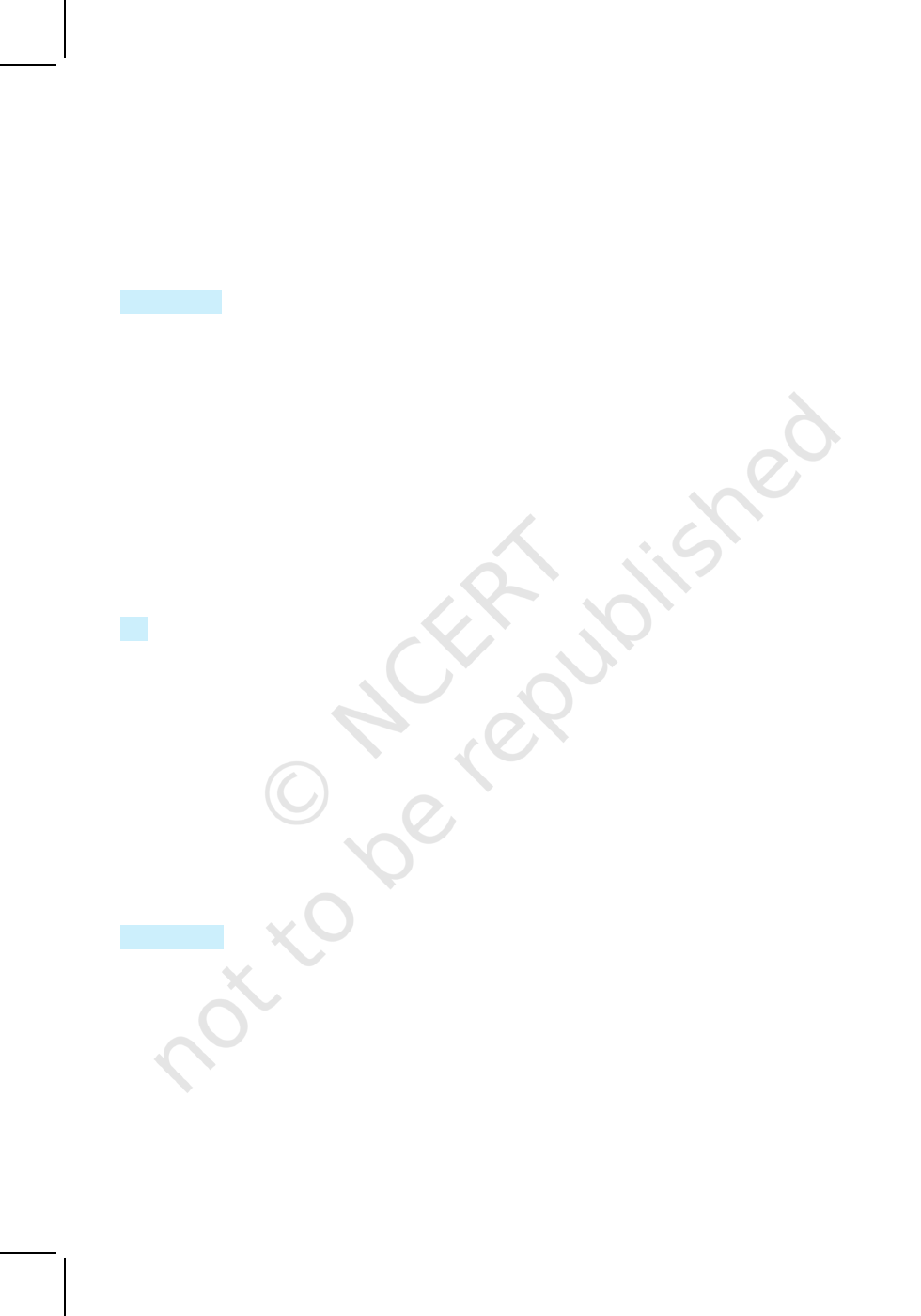6 MATHEMATICS
B = { x : x is a student presently studying in both Classes X and XI }
We observe that a student cannot study simultaneously in both Classes X and XI.
Thus, the set B contains no element at all.
Definition 1
A set which does not contain any element is called the empty set or the
null set or the
void set
.
According to this definition, B is an empty set while A is not an empty set. The
empty set is denoted by the symbol φ or { }.
We give below a few examples of empty sets.
(i) Let A = {
x : 1 < x < 2, x is a natural number}. Then A is the empty set,
because there is no natural number between 1 and 2.
(ii) B = {x : x
2
– 2 = 0 and x is rational number}. Then B is the empty set because
the equation x
2
– 2 = 0 is not satisfied by any rational value of x.
(iii) C = {x : x is an even prime number greater than 2}.Then C is the empty set,
because 2 is the only even prime number.
(iv) D = { x : x
2
= 4,
x is odd }. Then D is the empty set, because the equation
x
2
= 4 is not satisfied by any odd value of x.
1.4 Finite and Infinite Sets
Let A = {1, 2, 3, 4, 5}, B = {a, b, c, d, e, g}
and C = { men living presently in different parts of the world}
We observe that A contains 5 elements and B contains 6 elements. How many elements
does C contain? As it is, we do not know the number of elements in C, but it is some
natural number which may be quite a big number. By number of elements of a set S,
we mean the number of distinct elements of the set and we denote it by n (S). If n (S)
is a natural number, then S is non-empty finite set.
Consider the set of natural numbers. We see that the number of elements of this
set is not finite since there are infinite number of natural numbers. We say that the set
of natural numbers is an infinite set. The sets A, B and C given above are finite sets
and n(A) = 5, n(B) = 6 and n(C) = some finite number.
Definition 2 A set which is empty or consists of a definite number of elements is
called finite otherwise, the set is called infinite.
Consider some examples :
(i) Let W be the set of the days of the week. Then W is finite.
(ii) Let S be the set of solutions of the equation x
2
–16 = 0. Then S is finite.
(iii) Let G be the set of points on a line. Then G is infinite.
When we represent a set in the roster form, we write all the elements of the set
within braces { }. It is not possible to write all the elements of an infinite set within
braces { } because the numbers of elements of such a set is not finite. So, we represent
2020-21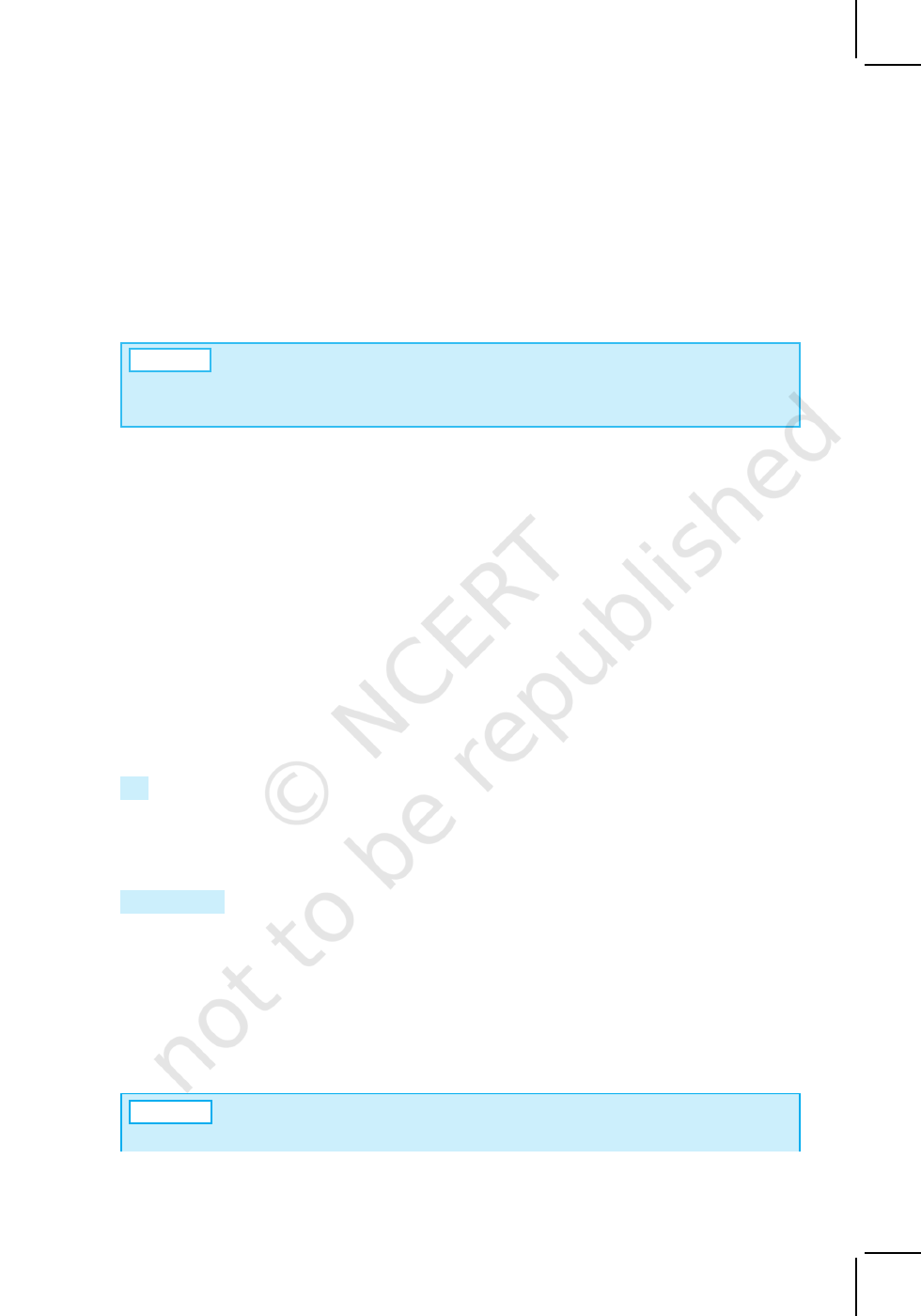SETS 7
some infinite set in the roster form by writing a few elements which clearly indicate the
structure of the set followed ( or preceded ) by three dots.
For example, {1, 2, 3 . . .} is the set of natural numbers, {1, 3, 5, 7, . . .} is the set
of odd natural numbers, {. . .,–3, –2, –1, 0,1, 2 ,3, . . .} is the set of integers. All these
sets are infinite.
A
Note All infinite sets cannot be described in the roster form. For example, the
set of real numbers cannot be described in this form, because the elements of this
set do not follow any particular pattern.
Example 6 State which of the following sets are finite or infinite :
(i) {
x : x N and (x – 1) (x –2) = 0}
(ii) {
x : x N and
x
2
= 4}
(iii) {
x : x N and 2
x –1 = 0}
(iv) {x : x N and x is prime}
(v) {x : x N and x is odd}
Solution (i) Given set = {1, 2}. Hence, it is finite.
(ii) Given set = {2}. Hence, it is finite.
(iii) Given set = φ. Hence, it is finite.
(iv) The given set is the set of all prime numbers and since set of prime
numbers is infinite. Hence the given set is infinite
(v) Since there are infinite number of odd numbers, hence, the given set is
infinite.
1.5 Equal Sets
Given two sets A and B, if every element of A is also an element of B and if every
element of B is also an element of A, then the sets A and B are said to be equal.
Clearly, the two sets have exactly the same elements.
Definition 3 Two sets A and B are said to be equal if they have exactly the same
elements and we write A = B. Otherwise, the sets are said to be unequal and we write
A B.
We consider the following examples :
(i) Let A = {1, 2, 3, 4} and B = {3, 1, 4, 2}. Then A = B.
(ii) Let A be the set of prime numbers less than 6 and P the set of prime factors
of 30. Then A and P are equal, since 2, 3 and 5 are the only prime factors of
30 and also these are less than 6.
A
Note A set does not change if one or more elements of the set are repeated.
For example, the sets A = {1, 2, 3} and B = {2, 2, 1, 3, 3} are equal, since each
2020-21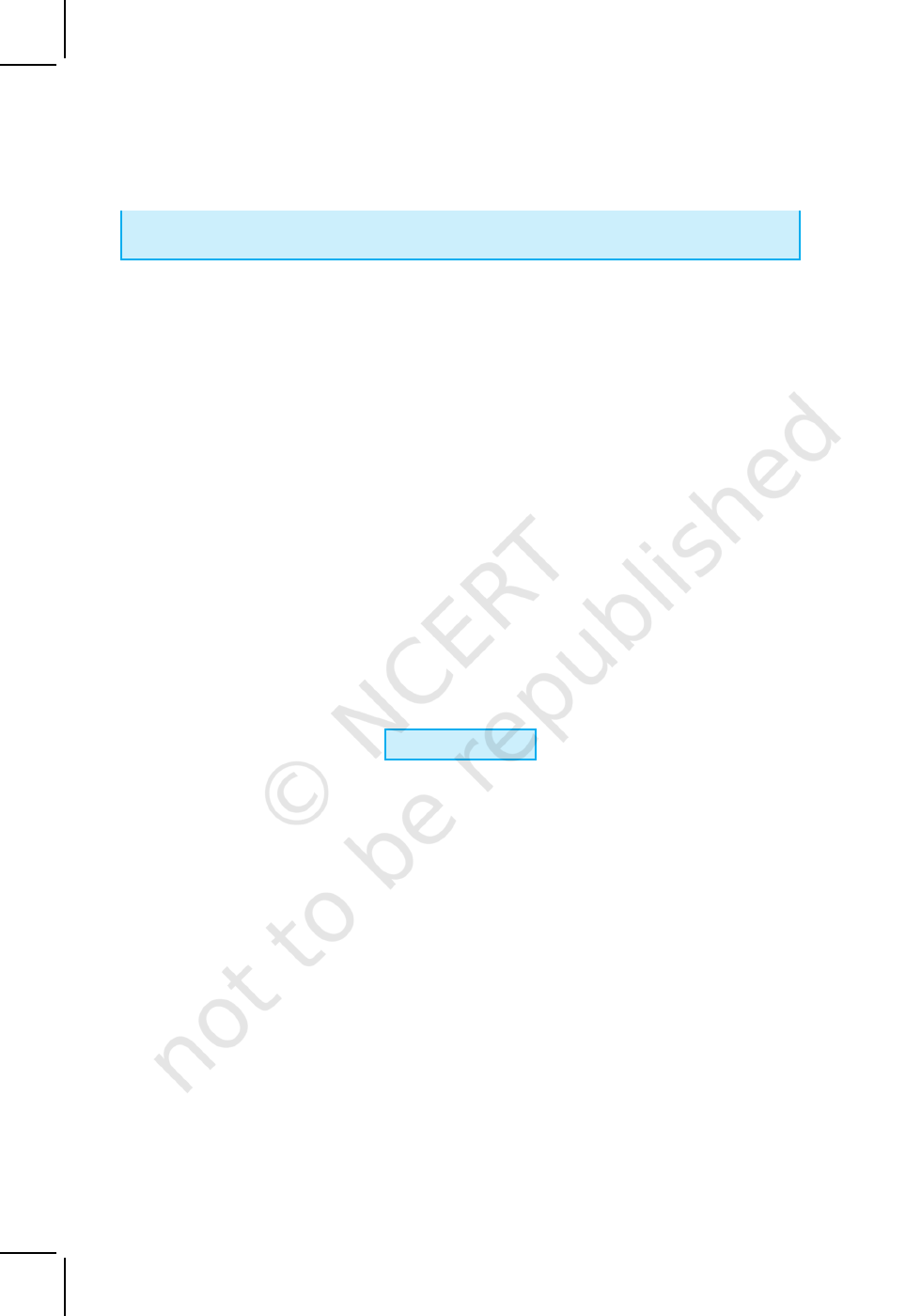8 MATHEMATICS
element of A is in B and vice-versa. That is why we generally do not repeat any
element in describing a set.
Example 7 Find the pairs of equal sets, if any, give reasons:
A = {0}, B = {
x : x > 15 and x < 5},
C = {x : x – 5 = 0 }, D = {
x: x
2
= 25},
E = {x : x is an integral positive root of the equation x
2
– 2x –15 = 0}.
Solution
Since 0
A and 0 does not belong to any of the sets B, C, D and E, it
follows that, A
B, A C, A D, A E.
Since B = φ but none of the other sets are empty. Therefore B C, B D
and B E. Also C = {5} but –5 D, hence C D.
Since E = {5}, C = E. Further, D = {–5, 5} and E = {5}, we find that, D E.
Thus, the only pair of equal sets is C and E.
Example 8 Which of the following pairs of sets are equal? Justify your answer.
(i) X, the set of letters in “ALLOY” and B, the set of letters in “LOYAL”.
(ii) A = {n : n Z and n
2
4} and B = {x : x R and x
2
– 3x + 2 = 0}.
Solution
(i) We have, X = {A, L, L, O, Y}, B = {L, O, Y, A, L}. Then X and B are
equal sets as repetition of elements in a set do not change a set. Thus,
X = {A, L, O, Y} = B
(ii) A = {–2, –1, 0, 1, 2}, B = {1, 2}. Since 0 A and 0 B, A and B are not equal sets.
EXERCISE 1.2
1. Which of the following are examples of the null set
(i) Set of odd natural numbers divisible by 2
(ii) Set of even prime numbers
(iii) { x : x is a natural numbers, x < 5 and x > 7 }
(iv) { y : y is a point common to any two parallel lines}
2. Which of the following sets are finite or infinite
(i) The set of months of a year
(ii) {1, 2, 3, . . .}
(iii) {1, 2, 3, . . .99, 100}
(iv) The set of positive integers greater than 100
(v) The set of prime numbers less than 99
3. State whether each of the following set is finite or infinite:
(i) The set of lines which are parallel to the x-axis
(ii) The set of letters in the English alphabet
(iii) The set of numbers which are multiple of 5
2020-21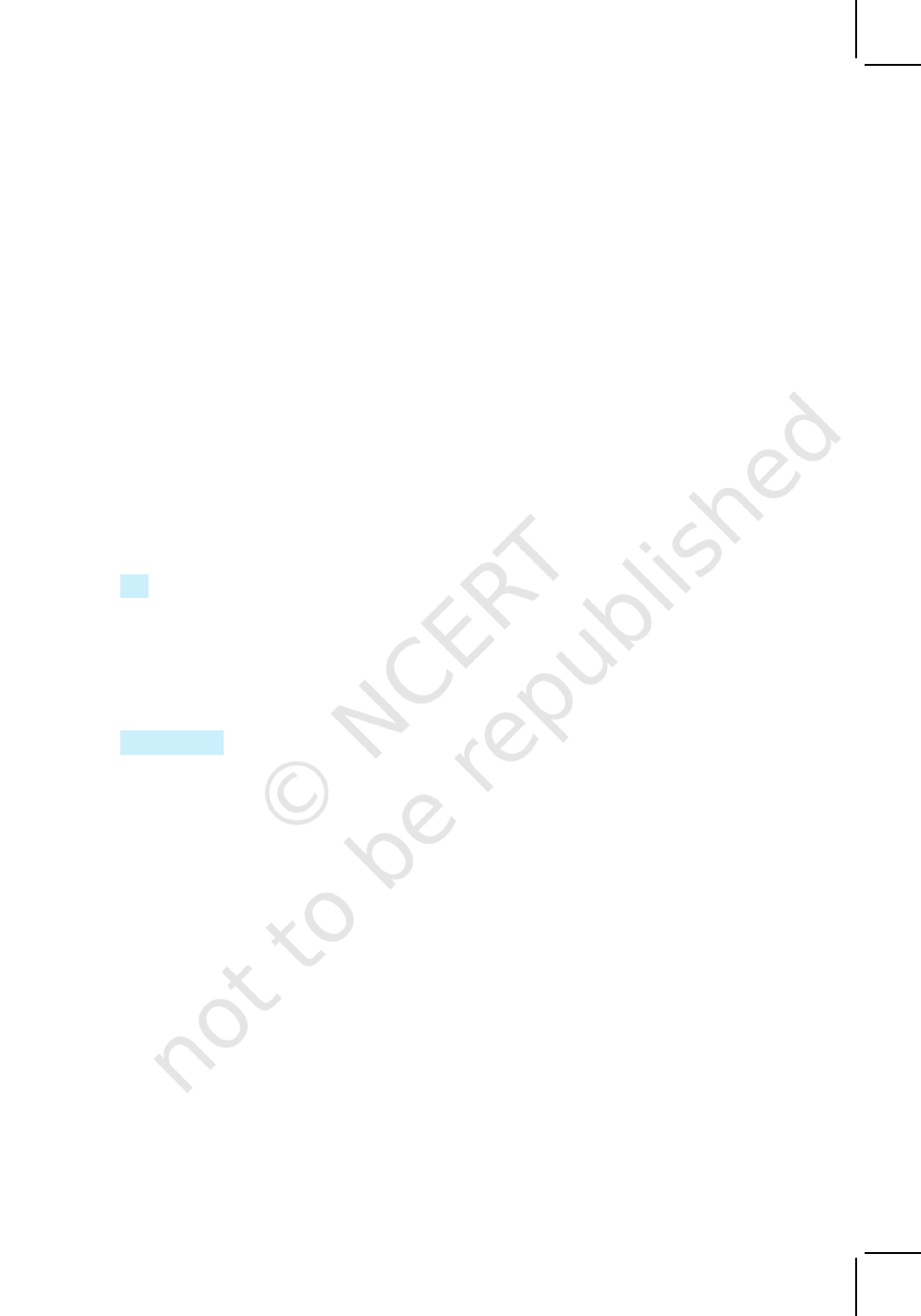SETS 9
(iv) The set of animals living on the earth
(v) The set of circles passing through the origin (0,0)
4. In the following, state whether A = B or not:
(i) A = { a, b, c, d } B = { d, c, b, a }
(ii) A = { 4, 8, 12, 16 } B = { 8, 4, 16, 18}
(iii) A = {2, 4, 6, 8, 10} B = {
x : x is positive even integer and x 10}
(iv) A = { x : x is a multiple of 10}, B = { 10, 15, 20, 25, 30, . . . }
5. Are the following pair of sets equal ? Give reasons.
(i) A = {2, 3}, B = {x
: x is solution of x
2
+ 5x + 6 = 0}
(ii) A = { x : x is a letter in the word FOLLOW}
B = { y : y is a letter in the word WOLF}
6. From the sets given below, select equal sets :
A = { 2, 4, 8, 12}, B = { 1, 2, 3, 4}, C = { 4, 8, 12, 14}, D = { 3, 1, 4, 2}
E = {–1, 1}, F = { 0, a}, G = {1, –1}, H = { 0, 1}
1.6 Subsets
Consider the sets : X = set of all students in your school, Y = set of all students in your
class.
We note that every element of Y is also an element of X; we say that Y is a subset
of X. The fact that Y is subset of X is expressed in symbols as Y
X. The symbol
stands for ‘is a subset of or ‘is contained in’.
Definition 4
A set A is said to be a subset of a set B if every element of A is also an
element of B.
In other words, A B if whenever a A, then a
B. It is often convenient to
use the symbol “” which means implies. Using this symbol, we can write the definiton
of subset as follows:
A B if a
A a B
We read the above statement as A is a subset of B if a is an element of A
implies that a is also an element of B”. If A is not a subset of B, we write A B.
We may note that for A to be a subset of B, all that is needed is that every
element of A is in B. It is possible that every element of B may or may not be in A. If
it so happens that every element of B is also in A, then we shall also have B A. In this
case, A and B are the same sets so that we have A B and B A A = B, where
” is a symbol for two way implications, and is usually read as if and only if (briefly
written as “iff”).
It follows from the above definition that every set A is a subset of itself, i.e.,
A A. Since the empty set φ has no elements, we agree to say that φ is a subset of
every set. We now consider some examples :
2020-21